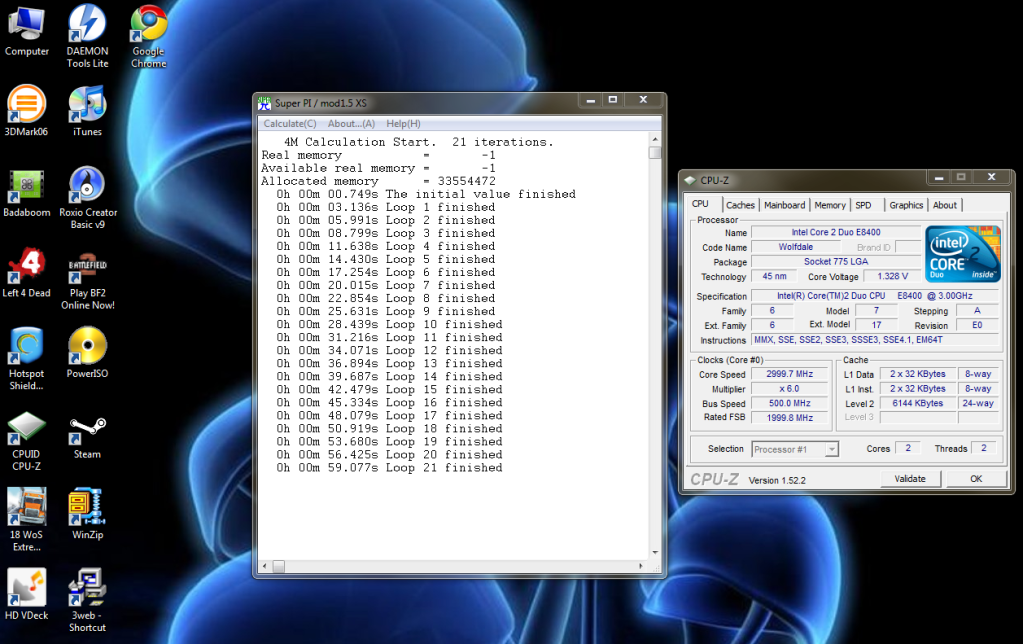# Thread: TBCS - Super Pi

1. ##Re: TBCS - Super PiReply With Quote

2. ##Re: TBCS - Super Pi

nice background! digital blasphemy ftw! lolReply With Quote

3. ##Re: TBCS - Super Pi

*bump*

I think I did it right....
Code:
```ammon@ammon-GA-78LMT-S2P:~/super_pi\$ ./pi 22
Version 2.0 of the super_pi for Linux OS
Fortran source program was translated into C program with version 19981204 of
f2c, then generated C source program was optimized manually.
pgcc 3.2-3 with compile option of "-fast -tp px -Mbuiltin -Minline=size:1000 -Mnoframe -Mnobounds -Mcache_align -Mdalign -Mnoreentrant" was used for the
compilation.
------ Started super_pi run : Sun Jan 29 17:04:03 MST 2012
Start of PI calculation up to 4194304 decimal digits
End of initialization. Time=       1.124 Sec.
I= 1 L=       0        Time=       2.996 Sec.
I= 2 L=       0        Time=       3.440 Sec.
I= 3 L=       1        Time=       3.436 Sec.
I= 4 L=       2        Time=       3.416 Sec.
I= 5 L=       5        Time=       3.432 Sec.
I= 6 L=      10        Time=       3.436 Sec.
I= 7 L=      21        Time=       3.512 Sec.
I= 8 L=      43        Time=       3.872 Sec.
I= 9 L=      87        Time=       3.704 Sec.
I=10 L=     174        Time=       3.620 Sec.
I=11 L=     349        Time=       3.532 Sec.
I=12 L=     698        Time=       3.460 Sec.
I=13 L=    1396        Time=       3.444 Sec.
I=14 L=    2794        Time=       3.592 Sec.
I=15 L=    5588        Time=       3.436 Sec.
I=16 L=   11176        Time=       3.600 Sec.
I=17 L=   22353        Time=       3.512 Sec.
I=18 L=   44707        Time=       3.416 Sec.
I=19 L=   89415        Time=       3.316 Sec.
I=20 L=  178831        Time=       3.248 Sec.
I=21 L=  357662        Time=       2.988 Sec.
End of main loop
End of calculation.    Time=      75.845 Sec.
End of data output.    Time=       0.368 Sec.
Total calculation(I/O) time=      76.213(       6.324) Sec.
------ Ended super_pi run : Sun Jan 29 17:05:20 MST 2012```Reply With Quote

####Posting Permissions

• You may not post new threads
• You may not post replies
• You may not post attachments
• You may not edit your posts
•